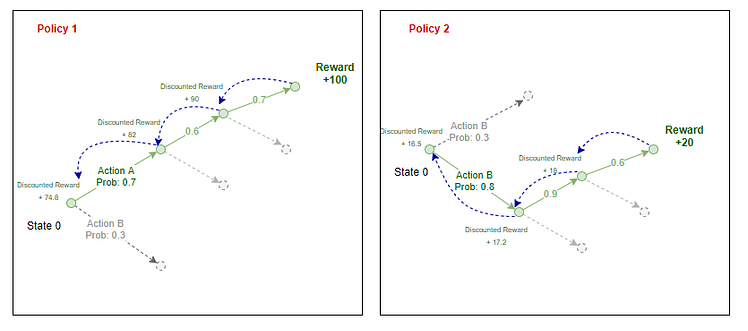Guest Blog — November 24, 2020

## Introduction

Lets’ solve OpenAI’s Cartpole, Lunar Lander, and Pong environments with REINFORCE algorithm.

Reinforcement learning is arguably the coolest branch of artificial intelligence. It has already proven its prowess: stunning the world, beating the world champions in games of Chess, Go, and even DotA 2.

In this article, I would be walking through a fairly rudimentary algorithm, and showing how even this can achieve a superhuman level of performance in certain games.

Reinforcement Learning deals with designing “Agents” that interacts with an “Environment” and learns by itself how to “solve” the environment by systematic trial and error. An environment could be a game like chess or racing, or it could even be a task like solving a maze or achieving an objective. The agent is the bot that performs the activity.

An agent receives “rewards” by interacting with the environment. The agent learns to perform the “actions” required to maximize the reward it receives from the environment. An environment is considered solved if the agent accumulates some predefined reward threshold. This nerd talk is how we teach bots to play superhuman chess or bipedal androids to walk.

## REINFORCE Algorithm

REINFORCE belongs to a special class of Reinforcement Learning algorithms called Policy Gradient algorithms. A simple implementation of this algorithm would involve creating a Policy: a model that takes a state as input and generates the probability of taking an action as output. A policy is essentially a guide or cheat-sheet for the agent telling it what action to take at each state. The policy is then iterated on and tweaked slightly at each step until we get a policy that solves the environment.

The policy is usually a Neural Network that takes the state as input and generates a probability distribution across action space as output.

The objective of the policy is to maximize the “Expected reward”.

Each policy generates the probability of taking an action in each station of the environment.

The agent samples from these probabilities and selects an action to perform in the environment. At the end of an episode, we know the total rewards the agent can get if it follows that policy. We backpropagate the reward through the path the agent took to estimate the “Expected reward” at each state for a given policy.

Here the discounted reward is the sum of all the rewards the agent receives in that future discounted by a factor Gamma.

The discounted reward at any stage is the reward it receives at the next step + a discounted sum of all rewards the agent receives in the future.The discounted factor is calculated for each state by back-propagating the rewards.

For the above equation this is how we calculate the Expected Reward:

As per the original implementation of the REINFORCE algorithm, the Expected reward is the sum of products of a log of probabilities and discounted rewards.

## Algorithm steps

The steps involved in the implementation of REINFORCE would be as follows:

1. Initialize a Random Policy (a NN that takes the state as input and returns the probability of actions)
2. Use the policy to play N steps of the game — record action probabilities-from policy, reward-from environment, action — sampled by agent
3. Calculate the discounted reward for each step by backpropagation
4. Calculate expected reward G
5. Adjust weights of Policy (back-propagate error in NN) to increase G
6. Repeat from 2

Check out the implementation using Pytorch on my Github.

## Demos

I have tested out the algorithm on Pong, CartPole, and Lunar Lander. It takes forever to train on Pong and Lunar Lander — over 96 hours of training each on a cloud GPU. There are several updates on this algorithm that can make it converge faster, which I haven’t discussed or implemented here. Checkout Actor-Critic models and Proximal Policy Optimization if interested in learning further.

CartPole

State:

Horizontal Position, Horizontal Velocity, Angle of the pole, Angular Velocity

Actions:

Push cart left, Push cart right

Random Policy Play:

Agent Policy Trained with REINFORCE:

## Lunar Lander

State:

The state is an array of 8 vectors. I am not sure what they represent.

Actions:

0: Do nothing

1: Fire Left Engine

2: Fire Down Engine

3: Fire Right Engine

Agent Policy Trained with REINFORCE:

## Pong

This was much harder to train. Trained on a GPU cloud server for days.

State: Image

Reinforcement Learning has progressed leaps and bounds beyond REINFORCE. My goal in this article was to 1. learn the basics of reinforcement learning and 2. show how powerful even such simple methods can be in solving complex problems. I would love to try these on some money-making “games” like stock trading … guess that’s the holy grail among data scientists.

Shoulders of giants:

3. Udacity’s reinforcement learning course (https://github.com/udacity/deep-reinforcement-learning)